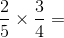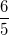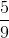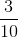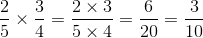# ISEE Middle Level Math : How to multiply fractions

## Example Questions

← Previous 1 3 4 5 6 7

### Example Question #336 : Numbers And Operations

Evaluate: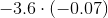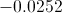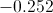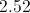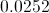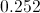Explanation:

The product of two negative numbers is the (positive) product of theie absolute values.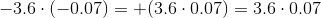There are a total of three digits to the right of the two decimal points. Therefore, the product can be calculated by multiplying 36 by 7, then placing the decimal point so that there are three digits at right.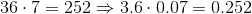This is the correct choice.

### Example Question #337 : Numbers And Operations

Solve: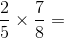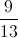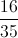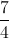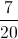Explanation: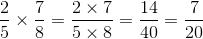### Example Question #338 : Numbers And Operations

Multiply: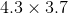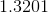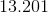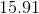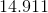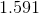Explanation:

First, multiply the numbers, ignoring the decimal points: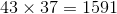Since the two factors in the original product have two digits to the right of the decimal points between them, position the decimal point in the product such that two digits are at its right. The result, therefore, is### Example Question #1 : Fractions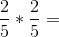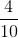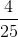Explanation:

Multiply the numerators and then denominators: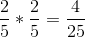### Example Question #340 : Numbers And Operations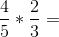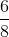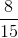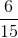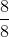Explanation:

Multiply the numerators then denominators: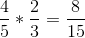Answer:### Example Question #1 : How To Multiply Fractions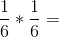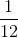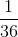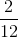Explanation:

Multiply the numerators and the denominators:Answer:### Example Question #2 : How To Multiply Fractions

What is the simplest form of the result: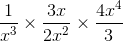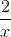Explanation:

In order to multiply fractions, we can simply multiply straight across: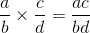So we can write: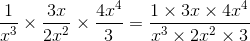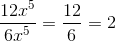### Example Question #3 : How To Multiply Fractions

Simplify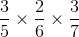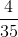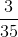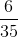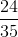Explanation:

In order to multiply fractions, we can simply multiply straight across:So we can write: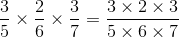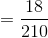Divide it by the least common factor (i.e. 6) to simplify: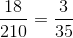### Example Question #1 : Fractions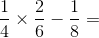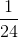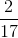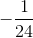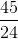Explanation:

In this problem we need to follow our order of operations; in this case we need to multiply before we subtract fractions.

To multiply fractions we just need to multiply the numerator by the numerator and the denominator by the denominator. In this case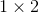(the numerators of the fractions being multiplied) and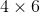(the denominators) giving us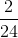We then rewrite our equation.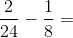In order to subtract fractions, we must have a common denominator. To do this we need to find a common multiple of 24 and 8. In this case, the 8 nicely goes into 24 three times, so we will only need to multiply one of our fractions to have common denominators.

To do this we must multiply one-eighth three times like this...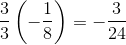Remember that if you are multiplying a fraction, you must multiply both the numerator and the denominator in order to obtain a multiple of your original factor.

Lastly subtract.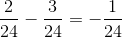### Example Question #5 : How To Multiply Fractions

Solve: